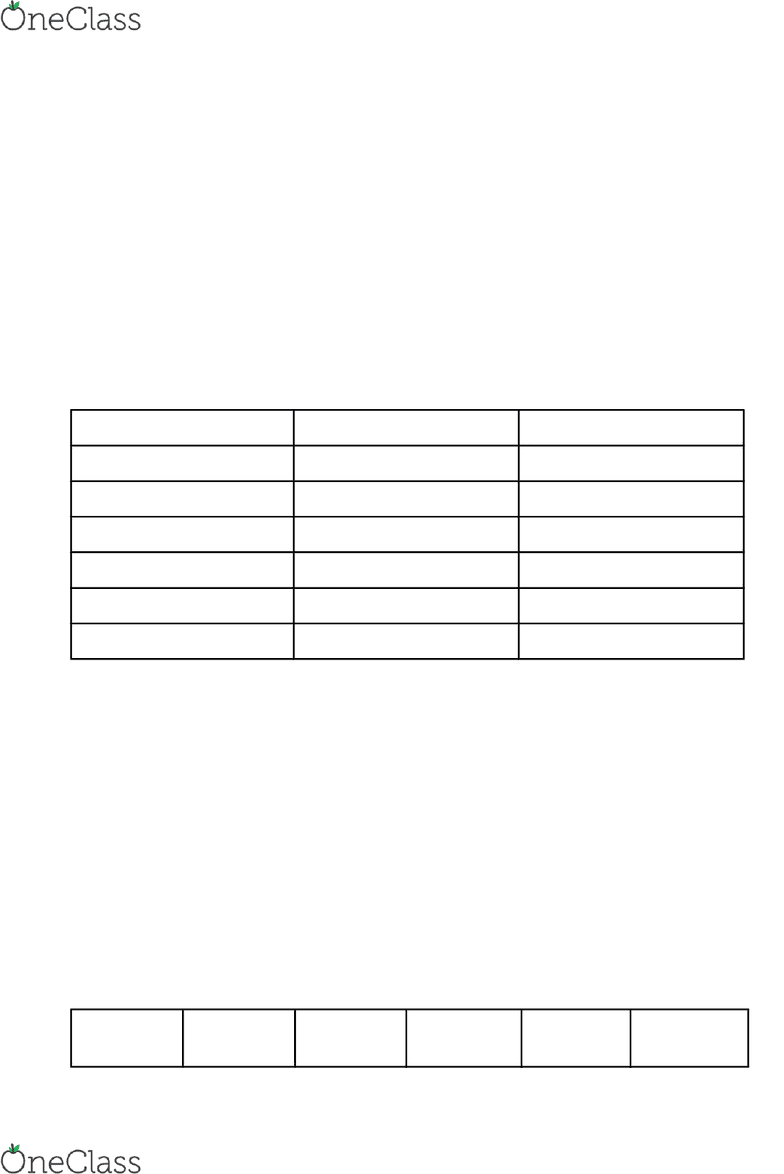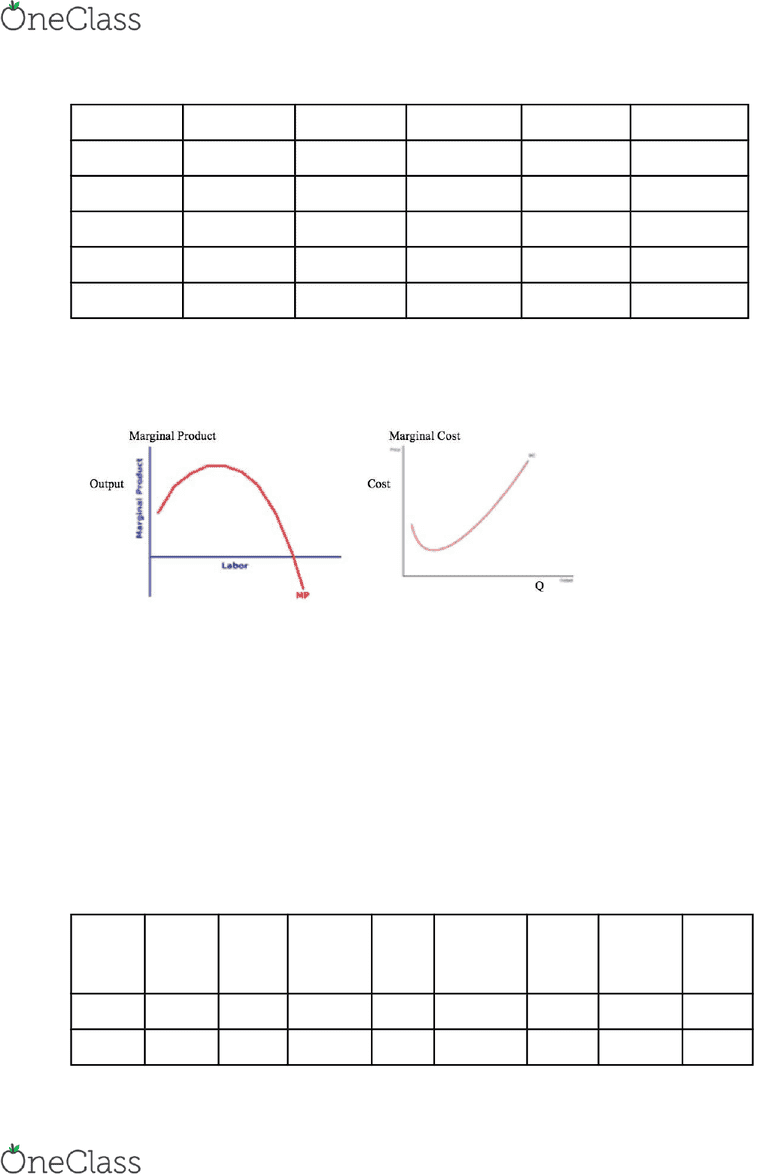# ECO101H1 Lecture Notes - Lecture 6: Average Variable Cost, Diminishing Returns, Marginal Cost

375 views5 pages
School
UTSG
Department
Economics
Course
ECO101H1
ProfessorKey question: how do firms maximize profits?
First step: understand firms’ cost schedules
Production function: Relates output to quantity of inputs (labour, capital)
Short-run: One input (capital) is fixed, while one input (labour) can vary
Long-run: All inputs (capital, labour) can vary
Example: General Motors
Short-run: GM can vary amount of labour (overtime, lay-offs)
GM cannot vary number of plants (capital)
Long-run: GM can vary number of plants and amount of labour
Production Schedules (Short-Run)
Total Product (TP): Total output, given labour input
Marginal Product* (MP): Increase in total output divided by increase in labour input
Law of Diminishing Returns: the marginal product of a variable input, in the presence of a
fixed input, eventually diminishes
Labour Output (Total Product) Marginal Product
0 0 --
1 4 4
2 10 6
3 13 3
4 15 2
5 16 1
Law of Diminishing Returns: Intuition
Fixed input: land (100 acres)
Variable input: labour (farm workers)
As number of farm workers increases, the amount of land available to each worker falls, and
Marginal Product of additional farm workers eventually falls
Measures of a Farm’s Costs of Production:
Total Cost (TC): Total of all costs
Total Fixed Cost (TFC): Total Cost of fixed input
Total Variable Cost (TVC): Total Cost of variable input
TC = TFC + TVC
**Marginal Cost (MC): Increase in total cost / increase in output
MC = change in TC / change in output
Wage rate: \$10
Labour (L) Output (Q) Fixed (TFC) Variable
(TVC)
Total (TC) Marginal (\$
per unit) (MC)
find more resources at oneclass.com
find more resources at oneclass.com
Unlock document

This preview shows pages 1-2 of the document.
Unlock all 5 pages and 3 million more documents.0 0 100 0 100 --
1 15 100 10 110 0.67
2 34 100 20 120 0.53
3 48 100 30 130 0.71
4 60 100 40 140 0.83
5 62 100 50 150 5.00
Observations
1. Law of Diminishing Returns (short-run) MP eventually decreases
2. MP increases => MC must be decreasing
MP decreases (eventually) => MC must be increasing (eventually)
Key Diagram #1
Measures of Average Costs
ATC (Average Total Cost) ATC = TC / Q
AVC (Average Variable Cost) AVC = TVC / Q
AFC (Average Fixed Cost) AFC = TFV / Q
ATC = AVC + AFC
Labour
(L)
Output
(Q)
Fixed
(TFC)
Variable
(TVC)
Total
(TC)
Marginal
(\$ per
unit) (MC)
Fixed
(AFC)
Variable
(AVC)
Total
(ATC)
0 0 100 0 100 -- -- -- --
1 15 100 10 110 0.67 6.67 0.67 7.33
find more resources at oneclass.com
find more resources at oneclass.com
Unlock document

This preview shows pages 1-2 of the document.
Unlock all 5 pages and 3 million more documents.

## Document Summary

Production function: relates output to quantity of inputs (labour, capital) Short-run: one input (capital) is fixed, while one input (labour) can vary. Short-run: gm can vary amount of labour (overtime, lay-offs) Long-run: gm can vary number of plants and amount of labour. Total product (tp): total output, given labour input. Marginal product* (mp): increase in total output divided by increase in labour input. Law of diminishing returns: the marginal product of a variable input, in the presence of a fixed input, eventually diminishes. As number of farm workers increases, the amount of land available to each worker falls, and. Marginal product of additional farm workers eventually falls. Total fixed cost (tfc): total cost of fixed input. Total variable cost (tvc): total cost of variable input. **marginal cost (mc): increase in total cost / increase in output. Mc = change in tc / change in output.